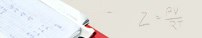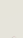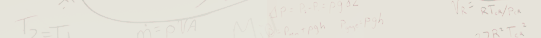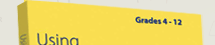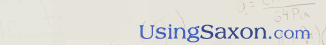Review the BookTable of ContentsJohn Saxon's LegacyYou Chose the Right BooksUse of CalculatorsGeometry CreditAbout the Author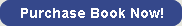Use of Calculators Back to Review BookAsk eighth or ninth grade students what the square root of 169, 196, or 225 is and you will be listening to a very long silence coupled with receiving an equally long blank stare from them. Then ask them what the product of 5280 x 5280 would be. Following their frantic search for the calculator you have hidden, you will find that without the calculator, most young students cannot even estimate the answer for you. The answer will be a bit larger than 25,000,000, but an estimate of 5 x 5 plus six zeros, would be a pretty close answer. This concept of using scientific notation to estimate the multiplication of large numbers was introduced in both Math 87 and Algebra ½. Checking our answer with a calculator, we would find the product of 5280 x 5280 to actually be 27,878,400. But what if students just blindly punched in the numbers, and left off a zero? How would they know that 2,787,840 is not the correct answer to the product of 5280 x 5280? When I approach parents and teachers with these questions, a student will invariably say to me, “But you're smarter than I am.” To which I reply, “I am not smarter than you, I am just older than you. And it is not that I do not like calculators; I do use one, but only when absolutely necessary.” Have them add the numbers 2386 + 3588 + 2986 and tell them, before they actually add them, to first “estimate” what the answer will be. Then have them add the numbers on paper and see if they added them correctly. Whether or not they use a calculator, their answer has to be a shade smaller than the sum of 24 + 36 + 30 + two more zeroes, or slightly smaller than 9000! I will wager the average eighth or ninth grade math student cannot estimate the process of either adding or multiplying large numbers. This is why John Saxon did not believe in the student using a calculator until the last twenty or so lessons in algebra one. And I agree with him! There are not too many problems in the last few lessons of algebra one that allows use of the calculator anyway. Actually, the scientific calculator is not really needed until lesson forty-three of Algebra 2, when trigonometric functions are first introduced. Letting students become familiar with a calculator late in Algebra 1 , however , makes using one easier when they get to Algebra 2. There is a certain satisfaction in being able to estimate the result of multiplying 5,500,310 times 2,206,213 without a calculator, and then, taking pencil and paper, seeing how close you came. I would estimate, as the Algebra ½ student should also, that the answer will be slightly larger than (6 x 2) followed by twelve zeroes. Another problem that arises for students using a scientific calculator too early in mathematics is the damage done as they learn where and how to use the fraction key on the calculator before they know how to find common denominators correctly! There is a fraction key found on modern scientific calculators. This key enables students using one of them to add two fractions with different denominators without first having to find their common denominator. The calculator does it for them! Now what do those young students do when, later in an algebra one course, they encounter the addition of two algebraic fractions? When they encounter a/b + c/d, not having learned how to calculate common denominators with numbered fractions, they will not have the faintest idea of how to acquire a common denominator using lettered variables. I am sadly reminded of the fourth grade math teacher who, when asked about the difficulty in teaching fractions to fourth graders, wailed at me, “I have neither the time nor the patience to teach them fractions. I give them a calculator and show them how to use the fraction key.” Years later, I had some of her students in my Algebra 1 class. They were failing because they could not decipher simple algebraic functions which required using common denominators. A good quarterback needs to be able to think fast and make correct decisions in fractions of a second. Mental manipulation of numbers or use of pen and paper to quickly multiply or divide trains the mind to do what good quarterbacking requires. We cripple our students when allowing them to use calculators at young ages and deny them the confidence that comes with fast, accurate thinking. Like the slide rules we carried to engineering classes more than fifty years ago, the calculator is but a tool to help the student speed up the tedious process of data manipulation to determine the correct answer. The student should command the calculator, not the other way around. I recently read an article that said a university had found that the old slide rule we used was not as slow as originally thought. They had tested the slide rule against a computer calculating the same data, and they found an engineer using the slide rule was capable of calculating some engineering formulas faster than the computer. An interesting observation I made was that most math teachers today no longer remember or teach what engineering notation is. We teach students only about scientific notation. We no longer teach them about engineering notation because we no longer use slide rules, for which the use of engineering notation was essential. When the time is right for the student to use a calculator, make sure it is a scientific calculator and not an adding machine. Regardless of how old the scientific calculator is, if it still works, use it! Scientific calculators will have keys labeled log, ln, cos, sin, and tan , on them. You can check to see if it is a scientific calculator by entering 2 + 4 x 3. If the answer is 14 , it is a scientific calculator. If the answer is 18 , it is not a scientific calculator. It does not contain the microchip that applies the order of operations used in mathematics. Oh, yes, I almost forgot. The square roots of 169, 196, and 225 are 13, 14 and 15 respectively! Home | Review the book | Purchase book | Testimonials | Newsletter | Contact Us © 2006 - 2019 AJ Publishers, LLC